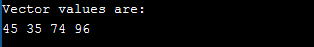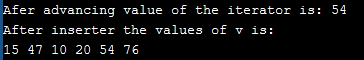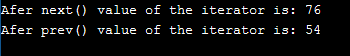# C++ Iterator: The Complete Guide

C++ Iterators are used to point at the memory addresses of STL containers. They are primarily used in the sequence of numbers, characters etc.used in C++ STL. It is like a pointer that points to an element of a container class (e.g., vector, list, map, etc.).

1. Operator * :
Returns the element of the current position.
2. Operator ++ and — :
It allows the iterator to step forward to the next element by ‘++,’ also it allows the iterator to step backward to the previous element by ‘–’
3. Operator == and !=:
Check whether two iterators represent the same position or not.
4. Operator =: Assigns an iterator (the position of the element to which it refers.

1. The STL provides iterators as a convenient abstraction for accessing many different types of containers.
2. Iterators for template classes that are generated inside a class scope with the syntax.

Example: class_name<parameters>::iterator

1. Iterators do not provide the bounds checking; it is possible to overstep the bounds of the container, resulting in the segmentation faults.
2. Different containers support different iterators, so it is not always possible to change an underlying container type without making the changes to your code.
3. Iterators can be invalidated if an underlying container (the container being iterated over) is changed significantly.

## C++ Iterators Example

C++ iterator represents a specific position in a container. If we want to make it work, iterators have the following basic operations which are precisely the interface of ordinary pointers when they are used to iterator over the elements of an array.

Let’s see the types of iterator.

## #Types of iterator in C++

Depending upon the work, an iterator can be categorized into 5 types, they are:

1. Input: An input iterator is one that a program can use to read values from a container. Dereferencing an input iterator allows us to read a value from a container, but it does not allow us to alter the value.
2. Output: An output iterator is similar to an input iterator, except that dereferencing is guaranteed to allow a program to modify a value of container element but not to read it.
3. Forward Iterator: This type of iterator is higher in the hierarchy than the input and output iterator. It has all the functionality that the input and output iterator has, also, the extra feature is it can move to the forward direction on step at a time.
4. Bidirectional: This type of iterator has all the features of Forwarding iterator; also, it can move towards both directions, means to the forward and backward both.
5. Random access iterator: The most powerful iterator is a random access iterator. As the name suggests, it can access any element at any position of the container. The application of this type of iterator is sort(), binary search, etc.

## #Syntax of declaring an iterator

See the following syntax.

`container_type <parameter_list>::iterator iterator_name;`

Here container_type is which type of container you are using (vector, list, etc.) and then you need to include which parameter you want to iterate (int, string, etc.) and then you need to write on iterator name.

`vector<int>::iterator myIterator;`

## #Operations in an iterator

### #begin()

The above function is used to return the beginning position of any container. See the following syntax.

`container_name.begin();`

### #end()

This function returns the ending position of any container.

`container_name.end();`

NOTE: All the programs will run above the c++11 version.

```#include<bits/stdc++.h>
using namespace std;
int main()
{
vector<int>v={45,35,74,96};
//declaring iterator
vector<int>::iterator i;
cout<<"Vector values are:\n";
for(i=v.begin();i<v.end();i++)
cout<<*i<<" ";
return 0;
}
```

See the below output.This function advances the iterator by n element position from its current position. It accepts two arguments:

1. iterator_name
2. position (value of n)

See the following syntax.

`advance(iterator_name, position)`

### #inserter()

The inserter() function is used to insert the element at any position in the container.

This function also accepts two arguments:

1. Container_name
2. The position where to insert

See the following syntax.

`inserter(container_name, position)`

See the following example.

```#include<bits/stdc++.h>
using namespace std;
int main()
{
vector<int> v={15,47,54,76};
//declaring iterator
vector<int>::iterator i=v.begin();
//to the point 3
cout<<"Afer advancing value of the iterator is: "<<*i;

//Now we will insert some values in v using inserter
vector<int>v1={10,20};
//this 10 and 20 will be inserted after 54
copy(v1.begin(), v1.end(), inserter(v,i));

cout<<"\nAfter inserter the values of v is:\n";
for(i=v.begin();i<v.end();i++)
cout<<*i<<" ";
return 0;
}
```

Okay, now see the following output.### #next()

It returns the iterator pointing to the element that it would be pointing to advanced n positions.

See the following syntax.

`next(iterator_name, position)`

### #prev()

It returns the iterator pointing to the element that it would be pointing to advanced -n positions.

The syntax of the prev() function is the following.

```prev(iterator_name, position)
```

Now, see the following programming example.

```#include<bits/stdc++.h>
using namespace std;
int main()
{
vector<int> v={15,47,54,76,23};
//declaring iterator
vector<int>::iterator i=v.begin();
auto it=next(i,3);
cout<<"Afer next() value of the iterator is: "<<*it<<"\n";
i=v.end();
auto it=prev(i,3);
cout<<"Afer prev() value of the iterator is: "<<*it<<"\n";
return 0;
}
```

See the following output.Finally, C++ Iterators Example is over.

Categories C++

This site uses Akismet to reduce spam. Learn how your comment data is processed.